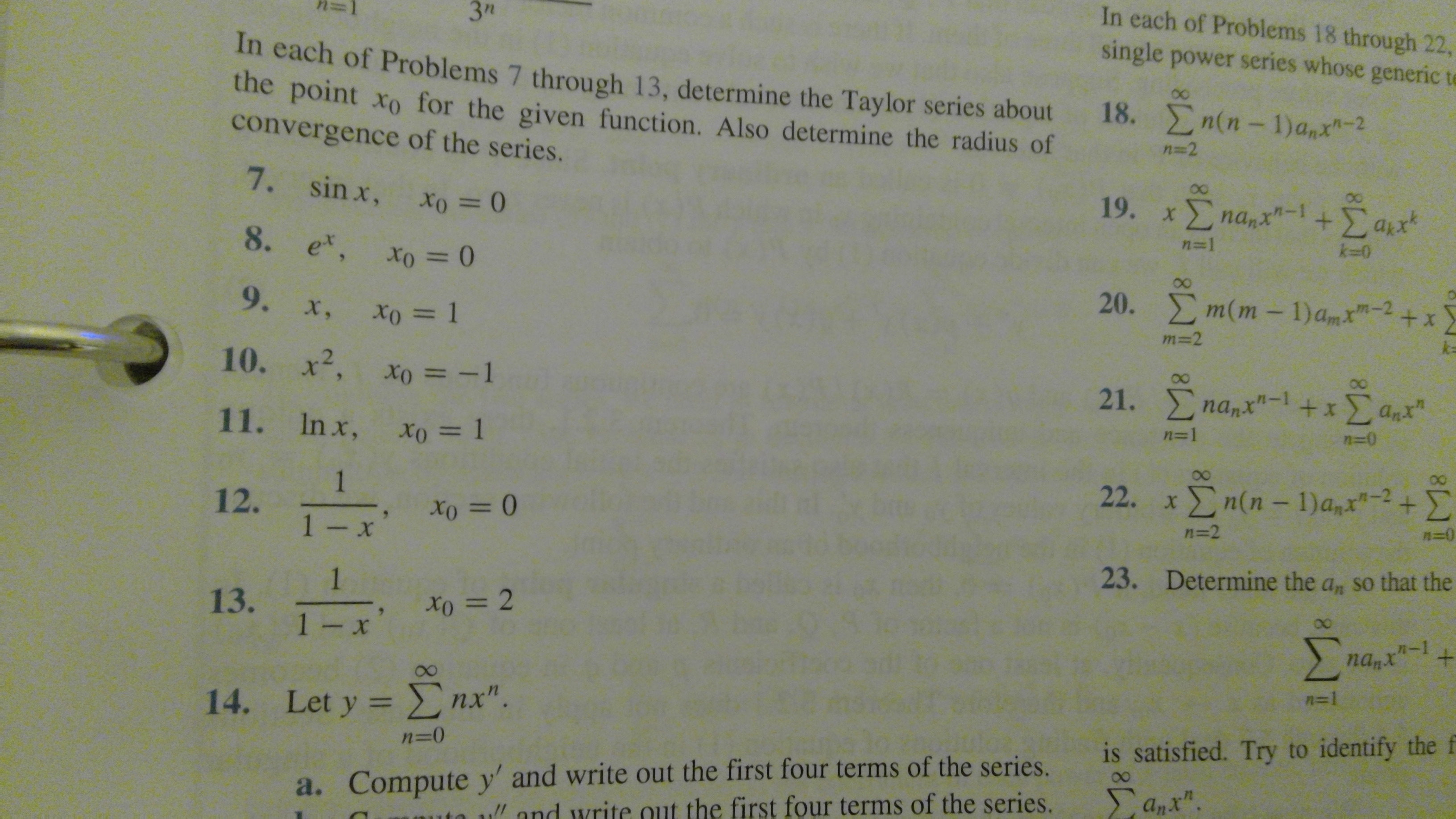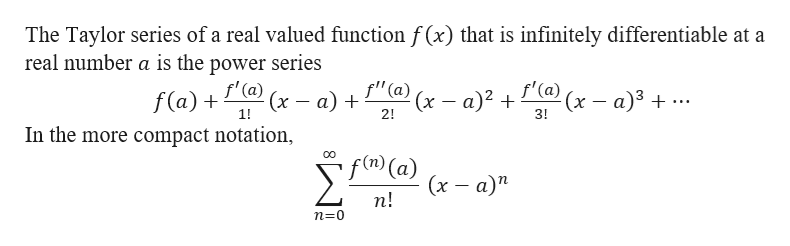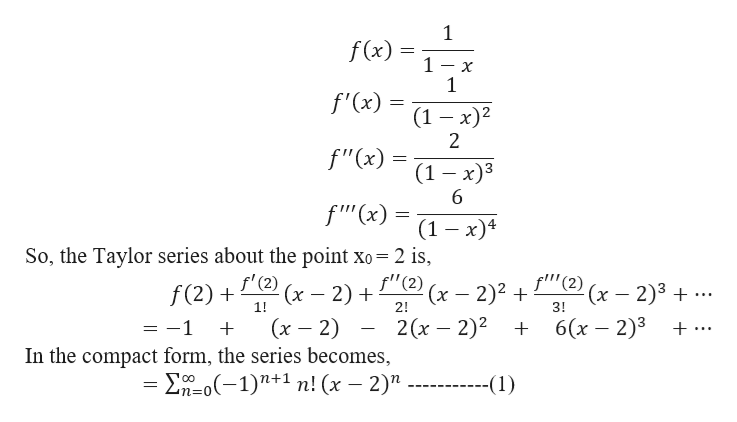# In each of Problems 18 through 22,3"single power series whose generic tIn each of Proble ms 7 through 13, determine the Taylor series aboutthe point xo for the given function. Also determine the radius ofconvergence of the series.0018. n(n-1) a,x-2n -27. sin x,19. xΣna,x"-1 Σ αx+Σαπ'n=1k-08. ex, xo = 0m(m - 1)am"-220.+ x9. x, xo = lm=2k=10. x2, xo = -121. nanx"-1 +xanxn=011. Inx, xo = 1n=122. xn(n-1)a,x"-2+112.1- xn=0=223.Determine the a, so that the113.1 - x+nan"-1n=114. Let y nx".is satisfied. Try to identify the fn=0a. Compute y' and write out the first four terms of the series.uand write out the first four terms of the series.Σaχ.E-E

Question
22 views

How do I appropriately answer number 13? This is a question from my Differential Equations course, and the name of the chapter is, "Review of Power Series." I think that to determine the radius of convergence is by doing some kind of root test, ratio test, etc.help_outlineImage TranscriptioncloseIn each of Problems 18 through 22, 3" single power series whose generic t In each of Proble ms 7 through 13, determine the Taylor series about the point xo for the given function. Also determine the radius of convergence of the series. 00 18. n(n-1) a,x-2 n -2 7. sin x, 19. xΣna,x"-1 Σ αx +Σαπ' n=1 k-0 8. ex, xo = 0 m(m - 1)am"-2 20. + x 9. x, xo = l m=2 k= 10. x2, xo = -1 21. nanx"-1 +xanx n=0 11. Inx, xo = 1 n=1 22. xn(n-1)a,x"-2+ 1 12. 1- x n=0 =2 23. Determine the a, so that the 1 13. 1 - x + nan"-1 n=1 14. Let y nx". is satisfied. Try to identify the f n=0 a. Compute y' and write out the first four terms of the series. uand write out the first four terms of the series. Σaχ. E-E fullscreen
check_circle

Step 1

We are given the function 1/(1-x). In this problem we need to find the Taylor series about the point x0 = 2. Also, we need to find the radius of convergence of the series formed.

Note:help_outlineImage TranscriptioncloseThe Taylor series of a real valued function f(x) that is infinitely differentiable at a real number a is the power series "(a) (x- a)2 f'(a) f'(a) (x - a) (х — а)3 + .. f(a) 1! 2! 3! In the more compact notation, 00 'f) (a) п! n-0 fullscreen
Step 2

Here,

...help_outlineImage Transcriptionclose1 f(x) 1- x 1 f'(x) (1 — х)2 2 f"(x) (1 — х)3 6 f'"'(x) (1 — х)4 So, the Taylor series about the point xo- 2 is, f'(2) f'"'(2) f"(2) (x- 2)2 +(x2)3 6(х — 2)3 (x 2) (x 2) In the compact form, the series becomes, 0(1)+1 n!(x 2)" f(2) 1! 2! 3! 2(х — 2)2 -1 + .. --(1) fullscreen

### Want to see the full answer?

See Solution

#### Want to see this answer and more?

Solutions are written by subject experts who are available 24/7. Questions are typically answered within 1 hour.*

See Solution
*Response times may vary by subject and question.
Tagged in

### Math